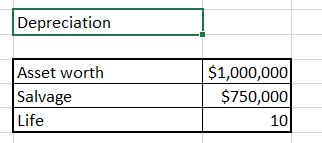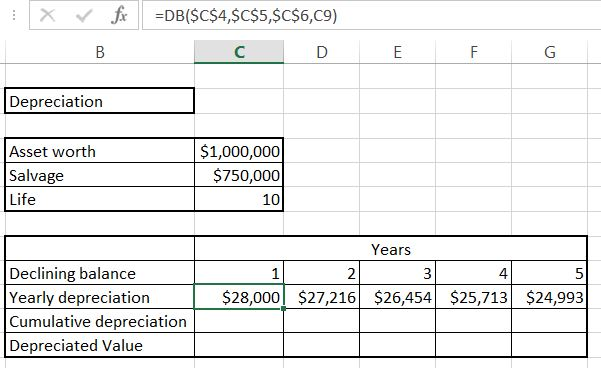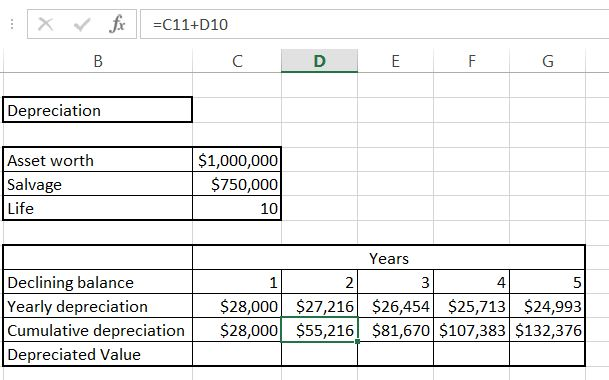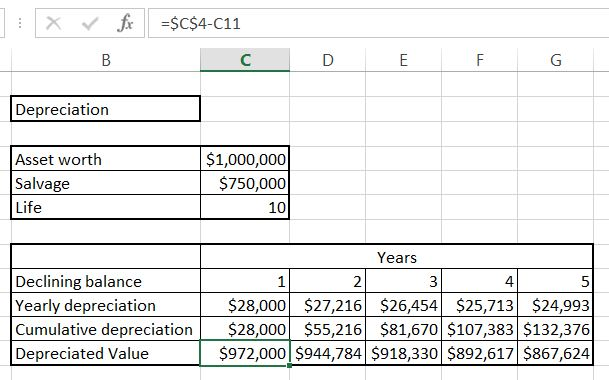#### How to Create a Declining Balance

A declining balance is a method of calculating the depreciation of an asset over time, where the depreciation rate remains constant, but the amount of depreciation decreases each period. In this Excel lesson you will learn yourself how to calculate Declining Balance Depreciation.

## Understanding Declining Balance Depreciation

The declining balance method is a type of accelerated depreciation, which means that it calculates a higher amount of depreciation in the early years of an asset’s life, and a lower amount of depreciation in the later years. This method is used to more accurately reflect the declining economic value of the asset over time.

To use the declining balance method in Excel, you need to have the original cost of the asset and the expected useful life of the asset. From there, you can calculate the depreciation rate, which is the rate at which the asset is depreciating over time.

Once you have the depreciation rate, you can calculate the depreciation expense for each period by multiplying the beginning balance of the asset by the depreciation rate. The beginning balance is then updated to reflect the remaining balance after the depreciation expense has been taken into account.

It’s important to note that the declining balance method assumes that the asset is depreciating at an accelerated rate, which may not be the case in reality. It is also a somewhat arbitrary method of calculating depreciation, and there are other methods, such as straight-line depreciation, that may be more appropriate for some assets.

Regardless of the method used, depreciation is an important concept in accounting and finance, as it provides a way of reflecting the loss in value of an asset over time and helps to accurately reflect the financial performance of a company.

## Data preparation

The asset:

• it is 1 million of dollars worth
• salvage value is \$750 000
• life of the asset is 10 yearsTo calculate a reducing balance we will use DB Excel formula.

## Formula syntax

Syntax of Declining Balance Excel formula is:

• cost
• salvage
• life
• period

Luckily, we already have all data we need.

## Declining Balance Calculations

To calculate yearly depreciation use =DB(\$C\$4,\$C\$5,\$C\$6,C9) formula.Absolute reference is needed because the data will not change in the table. Only the last arguments changes because it is the value of the year.

Cumulative depreciation is just =C11+D10. Drag it right to calculate all of them. This is just a current year value plus sum of previous ones.Deprecated value is =\$C\$4-C11 which is the starting value reduced by cumulative depreciation.This is Declining Balance formula and the easiest way to calculate Declining Balance Depreciation in Excel.